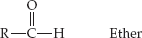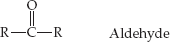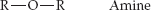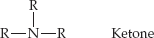×
Get Full Access to Introductory Chemistry - 5 Edition - Chapter 18 - Problem 83p
Get Full Access to Introductory Chemistry - 5 Edition - Chapter 18 - Problem 83p

×

# Based on its functional group, match the structure on the left with the correct name onISBN: 9780321910295 34

## Solution for problem 83P Chapter 18

Introductory Chemistry | 5th Edition

• Textbook Solutions
• 2901 Step-by-step solutions solved by professors and subject experts
• Get 24/7 help from StudySoup virtual teaching assistantsIntroductory Chemistry | 5th Edition

4 5 1 377 Reviews
26
5
Problem 83P

Problem 83P

Based on its functional group, match the structure on the left with the correct name on the right.

(a)(b)(c)(d)Step-by-Step Solution:

Solution 83P :

Step 1:

Here, based on the functional group, we should match the structure on the left with the correct name on the right.

Given :

(a)(b)(c)(d)Step 2 of 2

##### ISBN: 9780321910295

This textbook survival guide was created for the textbook: Introductory Chemistry, edition: 5. The answer to “Based on its functional group, match the structure on the left with the correct name on the right.(a) (b) (c) (d)” is broken down into a number of easy to follow steps, and 21 words. Introductory Chemistry was written by and is associated to the ISBN: 9780321910295. The full step-by-step solution to problem: 83P from chapter: 18 was answered by , our top Chemistry solution expert on 05/06/17, 06:45PM. This full solution covers the following key subjects: based, correct, functional, Group, its. This expansive textbook survival guide covers 19 chapters, and 2046 solutions. Since the solution to 83P from 18 chapter was answered, more than 351 students have viewed the full step-by-step answer.

Unlock Textbook Solution